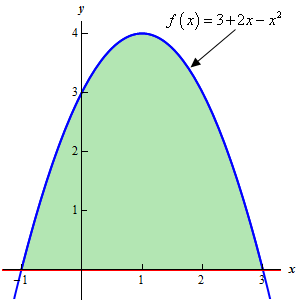Paul's Online Notes
Home / Calculus I / Applications of Integrals / Area Between Curves
Show Mobile Notice Show All Notes Hide All Notes
Mobile Notice
You appear to be on a device with a "narrow" screen width (i.e. you are probably on a mobile phone). Due to the nature of the mathematics on this site it is best views in landscape mode. If your device is not in landscape mode many of the equations will run off the side of your device (should be able to scroll to see them) and some of the menu items will be cut off due to the narrow screen width.

### Section 6.2 : Area Between Curves

1. Determine the area below $$f\left( x \right) = 3 + 2x - {x^2}$$ and above the $$x$$-axis.

Show All Steps Hide All Steps

Hint : It’s generally best to sketch the bounded region that we want to find the area of before starting the actual problem. Having the sketch of the graph will usually help with determining the upper/lower functions and the limits for the integral.
Start Solution

Let’s start off with getting a sketch of the region we want to find the area of.

We are assuming that, at this point, you are capable of graphing most of the basic functions that we’re dealing with in these problems and so we won’t be showing any of the graphing work here.

Here is a sketch of the bounded region we want to find the area of.Show Step 2

It should be clear from the graph that the upper function is the parabola (i.e. $$y = 3 + 2x - {x^2}$$) and the lower function is the $$x$$-axis (i.e. $$y = 0$$).

Since we weren’t given any limits on $$x$$ in the problem statement we’ll need to get those. From the graph it looks like the limits are (probably) $$- 1 \le x \le 3$$. However, we should never just assume that our graph is accurate or that we were able to read it accurately. For all we know the limits are close to those we guessed from the graph but are in fact slightly different.

So, to determine if we guessed the limits correctly from the graph let’s find them directly. The limits are where the parabola crosses the $$x$$-axis and so all we need to do is set the parabola equal to zero (i.e. where it crosses the line $$y = 0$$) and solve. Doing this gives,

$3 + 2x - {x^2} = 0\hspace{0.25in} \to \hspace{0.25in} - \left( {x + 1} \right)\left( {x - 3} \right) = 0\hspace{0.25in} \to \hspace{0.25in}x = - 1,\,\,\,\,x = 3$

So, we did guess correctly, but it never hurts to be sure. That is especially true here where finding them directly takes almost no time.

Show Step 3

At this point there isn’t much to do other than step up the integral and evaluate it.

We are assuming that you are comfortable with basic integration techniques so we’ll not be including any discussion of the actual integration process here and we will be skipping some of the intermediate steps.

The area is,

$A = \int_{{ - 1}}^{3}{{3 + 2x - {x^2}\,dx}} = \left. {\left( {3x + {x^2} - \frac{1}{3}{x^3}} \right)} \right|_{ - 1}^3 = \require{bbox} \bbox[2pt,border:1px solid black]{{\frac{{32}}{3}}}$